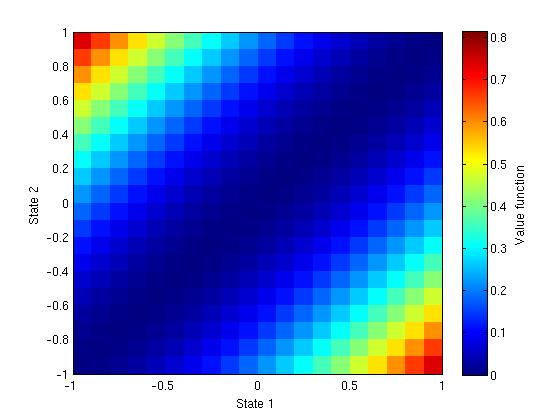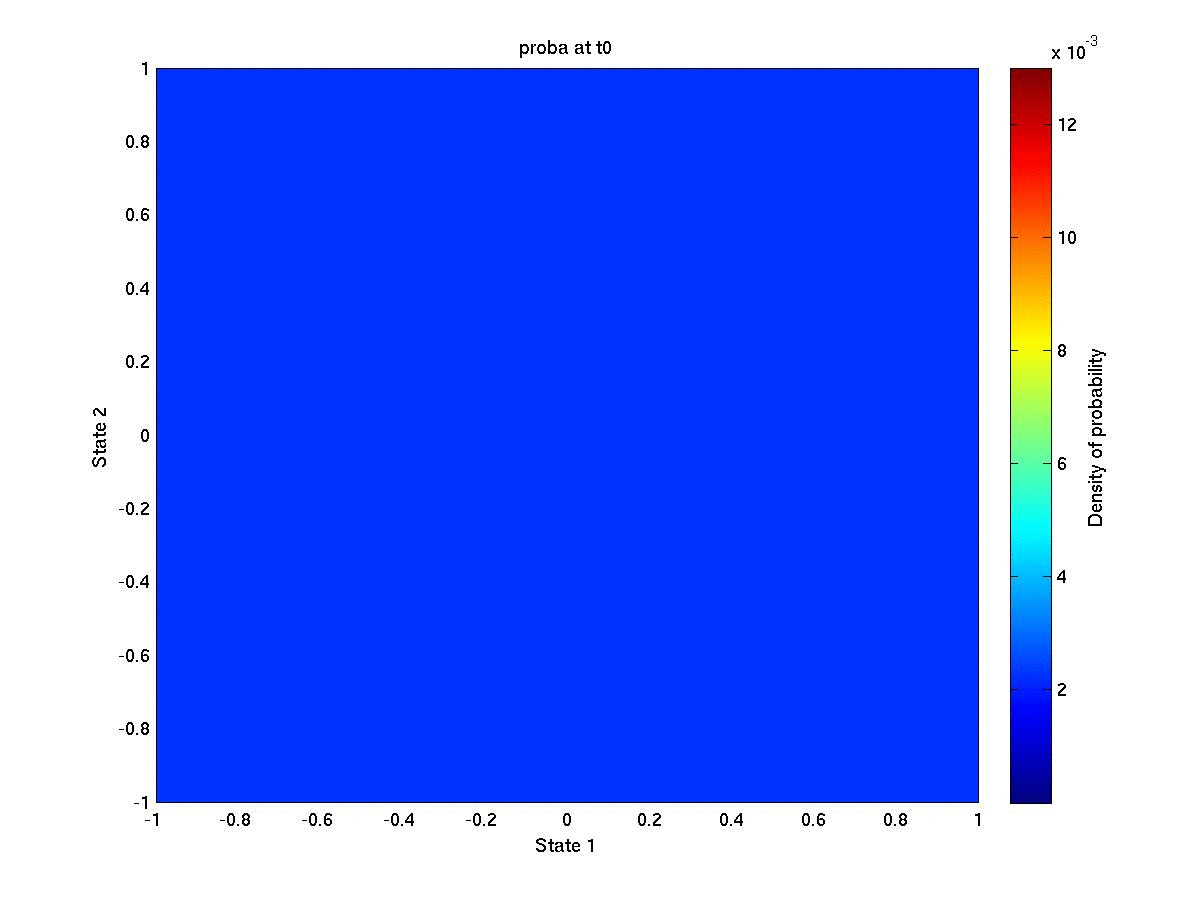# 2D Integrator

We illustrate the process law feature on a simple deterministic problem, without discrete modes. We consider a classical 2D integrator$Latex formula$

The figure shows the value function at initial time. As expected, the value function is 0 along the first bisector (x1 = x2) and increases symmetrically when getting farther form it.We set the state probabilities at initial time according to a discrete uniform distribution$Latex formula$

The following animated figure shows the evolution of the state probabilities over the grid for each time step. We observe a concentration of the distribution towards the first bisector. This is not surprising since every optimal trajectory tries to reach the bisector with minimal quadratic cost.